# 量子搜索算法 Grover search

## 问题定义：

Problem：

$f: \{ 0,1,2,3,……,N-1 \} \rightarrow \{0,1\}$

## 解法

### Grover search 算法

Grover search 算法一共分为两步：

1. Phase Inversion

Phase Inversion:

$\mu$ 是所有概率幅的平均值，$\mu= \frac{\sum_x \alpha_x}{N}$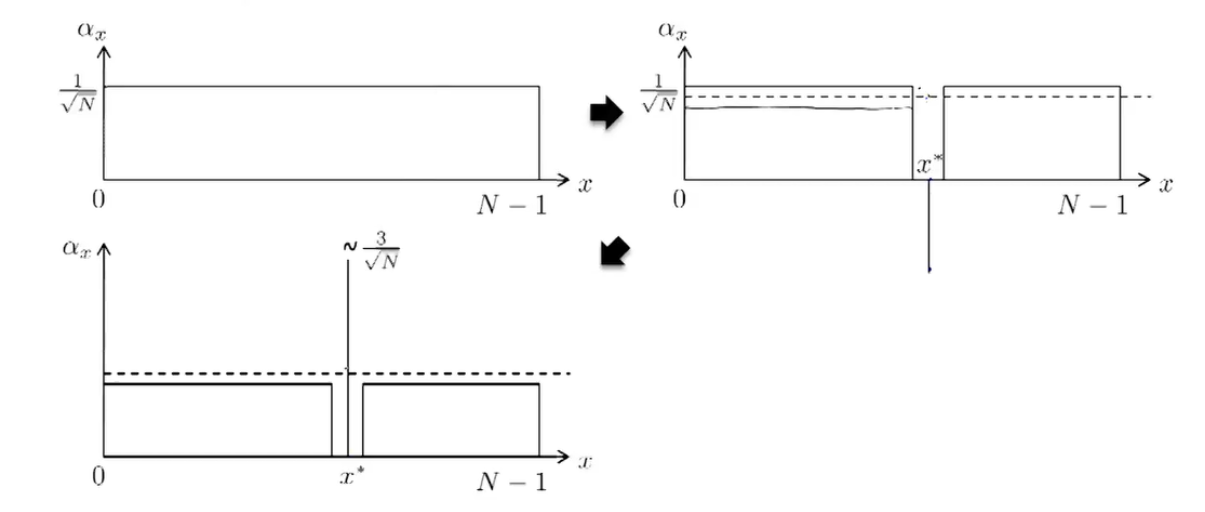## Phase Inversion:

$\sum_{x } \alpha_x|x\rangle$ 变成 $\sum_{x \neq x^*} \alpha_x|x\rangle -\alpha_{x^*}|x^*\rangle$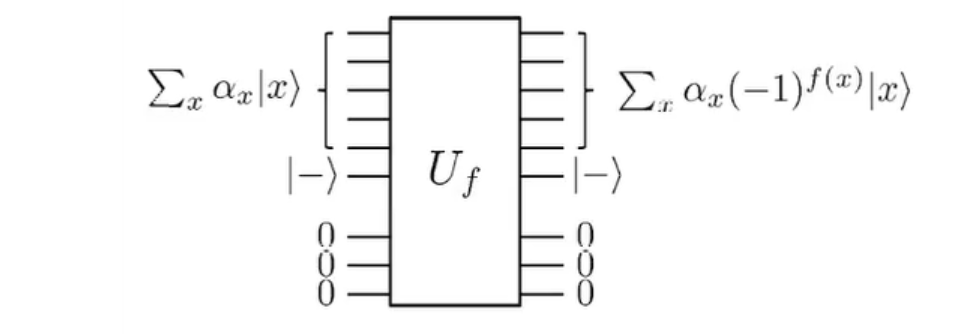$\alpha_x$ 变成 $2\mu- \alpha_x$ ，这个就要比前一个麻烦了

$\left[ \begin{array}{} 1 & 0 & …& 0 \\ 0 & -1 & …& 0 \\…\\0 & 0 & …& -1 \end{array}\right]\left[ \begin{array}{} a_0\\a_1\\…\\a_{n-1} \end{array}\right]=\left[ \begin{array}{} a_0\\-a_1\\…\\-a_{n-1} \end{array}\right]$

$|\mu\rangle$ 是一个怎样的态？

$\frac{1}{2^{\frac{n}{2}}} \sum_{x \in \{0,1 \}^n}|x\rangle$$|0\rangle$之间的相互转换，这就是我们最最熟悉的Hadamard Transform了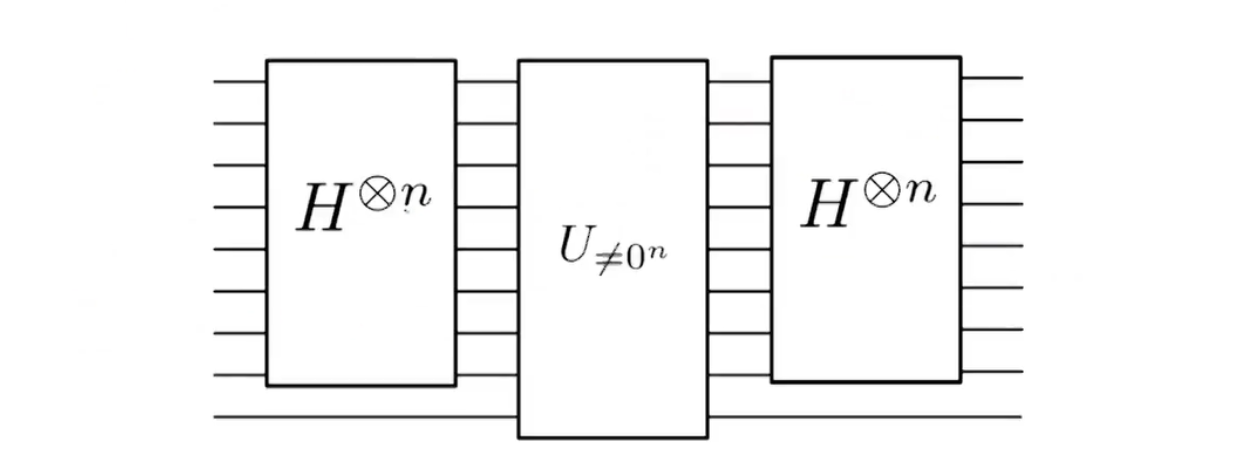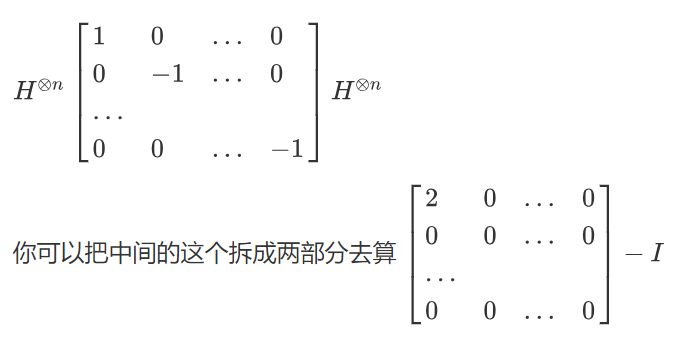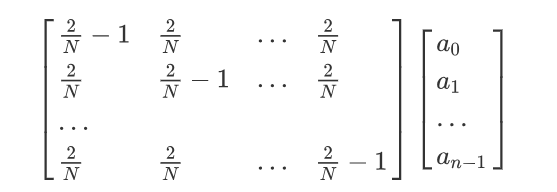$\frac{2}{N} \sum _{y=0}^{N} \alpha_y$ 恰好就是 $2\mu$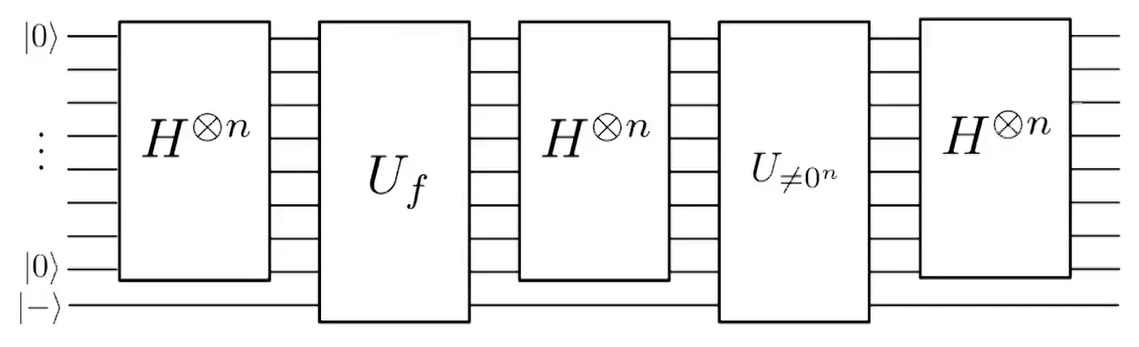Quantume Mechanics & Quantume Computation Lecture 11

posted @ 2019-08-17 18:05  夏天喵  阅读(4357)  评论(1编辑  收藏